# scipy.spatial.distance.jaccard¶

scipy.spatial.distance.jaccard(u, v)
Computes the Jaccard-Needham dissimilarity between two boolean

n-vectors u and v, which israc{c_{TF} + c_{FT}}
{c_{TT} + c_{FT} + c_{TF}}

where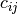is the number of occurrences of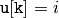and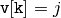for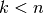.

Parameters : u : ndarray An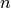-dimensional vector. v : ndarray An-dimensional vector. d : double The Jaccard distance between vectors u and v.

#### Previous topic

scipy.spatial.distance.hamming

#### Next topic

scipy.spatial.distance.kulsinski